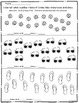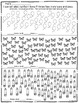# Place Value ~ How many tens and ones?Subject
Resource Type
File Type
PDF (17 MB|5 pages)
Standards
\$1.25
• Product Description
• Standards

Students practice using what they know about tens and ones to answer the question How Many? by finding groups of tens and ones instead of counting all.

Included are 3 sheets. Each sheet starts by asking the kids to find out how many by circling groups of tens. They then record how many tens and ones they have and how many that equals.

Sheet 1 has quantities less than 20.

Sheet 2 has quantities from 20-30.

Sheet 3 has quantities 35-70.

Check out...

Place Value ~ How many tens and ones? ~ Back To School Themed

Place Value ~ How many tens and ones? ~ Halloween

Place Value ~ How many tens and ones? ~ The Day of the Dead

Place Value ~ How many tens and ones? ~ Thanksgiving

Place Value ~ How many tens and ones? ~ Christmas

Place Value ~ How many tens and ones? ~ Valentine's Day

Place Value ~ How many tens and ones? ~ St. Patrick's Day

Place Value ~ How many tens and ones? ~ Earth Day

Place Value ~ How many tens and ones? ~ Easter

Don't forget to follow my store so you'll be notified of new and free products that I list.

As always, I appreciate your feedback and you'll earn valuable TPT credits to use in future purchases.

Thank you!

Understand that the two digits of a two-digit number represent amounts of tens and ones. Understand the following as special cases:
Total Pages
5 pages